####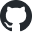https://github.com/rsharp-lang/R-sharp/tree/master/studio/RData## RData反序列化基础

### 链表访问

<Extension>
Public Function LinkVisitor(robj As RObject, key As String) As RObject
Dim tag As RObject

Do While Not robj Is Nothing
tag = robj.tag

If tag Is Nothing AndAlso robj.referenced_object Is Nothing Then
Return Nothing
End If

If tag.characters = key Then
Return robj
End If
If Not tag.referenced_object Is Nothing AndAlso tag.referenced_object.characters = key Then
Return robj
End If

robj = robj.value.CDR
Loop

Return Nothing
End Function

data = attrs.LinkVisitor("row.names")

### 字符串解码

<Extension>
Public Function DecodeCharacters(r_char As RObject) As String
If r_char.value Is Nothing Then
Return ""
ElseIf r_char.info.type = RObjectType.CHAR Then
Dim bytes As Byte() = DirectCast(r_char.value.data, Byte())
Dim encoding As Encoding = Encoding.UTF8

If r_char.info.gp And CharFlags.UTF8 Then
encoding = Encoding.UTF8
ElseIf r_char.info.gp And CharFlags.LATIN1 Then
encoding = Encoding.Latin1
ElseIf r_char.info.gp And CharFlags.ASCII Then
encoding = Encoding.ASCII
ElseIf r_char.info.gp And CharFlags.BYTES Then
encoding = Encoding.ASCII
End If

Return encoding.GetString(bytes)
Else
Return ""
End If
End Function

Public Enum CharFlags
HAS_HASH = 1
BYTES    = 1 << 1
LATIN1   = 1 << 2
UTF8     = 1 << 3
CACHED   = 1 << 5
ASCII    = 1 << 6
End Enum

### 向量读取

Public Class RStreamReader

<MethodImpl(MethodImplOptions.AggressiveInlining)>
Public Shared Function ReadString(robj As RObject) As String
Return robj.DecodeCharacters
End Function

<MethodImpl(MethodImplOptions.AggressiveInlining)>
Public Shared Function ReadNumbers(robj As RObject) As Double()
Return REnv.asVector(Of Double)(robj.value.data)
End Function

<MethodImpl(MethodImplOptions.AggressiveInlining)>
Public Shared Function ReadIntegers(robj As RObject) As Long()
Return REnv.asVector(Of Long)(robj.value.data)
End Function

<MethodImpl(MethodImplOptions.AggressiveInlining)>
Public Shared Function ReadLogicals(robj As RObject) As Boolean()
Return REnv.asVector(Of Boolean)(robj.value.data)
End Function

''' <summary>
''' read R vector in any element data type
''' </summary>
''' <param name="robj"></param>
''' <returns></returns>
Public Shared Function ReadVector(robj As RObject) As Array
Select Case robj.info.type
Case Else
Throw New NotImplementedException(robj.info.ToString)
End Select
End Function
End Class

• 对于CHAR向量，其真实数据为字符集合，在RData之中是以字节数组的形式存储，其也是STR向量的底层数据类型
• 对于STR向量，其为字符串集合，其建立在CHAR向量的基础上，STR向量是基于多个CHAR向量堆叠而成的向量数据。虽然STR向量有点类似于复合数据类型，但是在R环境中其任然被认为是一种不可分的基元类型

Friend Shared Function ReadStrings(robj As Object) As String()
If TypeOf robj Is RList Then
Dim rlist As RList = DirectCast(robj, RList)

Else
Return DirectCast(rlist.data, RObject()) _
.ToArray
End If

ElseIf DirectCast(robj, RObject).info.type = RObjectType.LIST Then
Else
Dim obj As RObject = DirectCast(robj, RObject)

If obj.info.type = RObjectType.STR Then
Return DirectCast(obj.value.data, RObject()) _
.ToArray
Else
End If
End If
End Function

## R数据类型反序列化

### 反序列化vector

' R storage units (nodes)
' Two types: SEXPREC(non-vectors) And VECTOR_SEXPREC(vectors)

' Node: VECTOR_SEXPREC
' The vector types are RAWSXP, CHARSXP, LGLSXP, INTSXP, REALSXP, CPLXSXP, STRSXP, VECSXP, EXPRSXP And WEAKREFSXP.
Friend ReadOnly elementVectorFlags As Index(Of RObjectType) = {
RObjectType.CPLX,
RObjectType.EXPR,
RObjectType.INT,
RObjectType.LGL,
RObjectType.RAW,
RObjectType.REAL,
RObjectType.CHAR,
RObjectType.STR,
RObjectType.WEAKREF
}

<Extension>
Private Function CreateRVector(robj As RObject) As vector
Dim type As RType = robj.info.GetRType
Dim factor As factor = If(RObjectSignature.HasFactor(robj), robj.attributes.value.CreateFactor, Nothing)
Dim vec As New vector(data, type) With {
.factor = factor
}

Return vec
End Function

factor值的读取，就是对一个字符串数组的读取操作：

<Extension>
Private Function CreateFactor(robj As RList) As factor
Dim data = robj.CAR
Dim factor As factor = factor.CreateFactor(levels, ordered:=True)

Return factor
End Function

strs = factor.asCharacter(vector)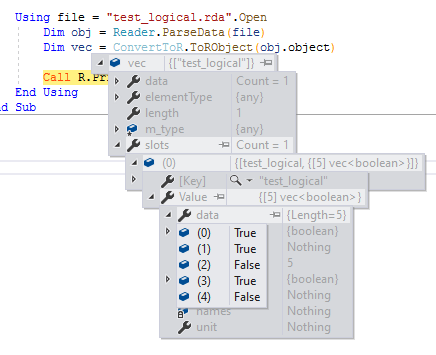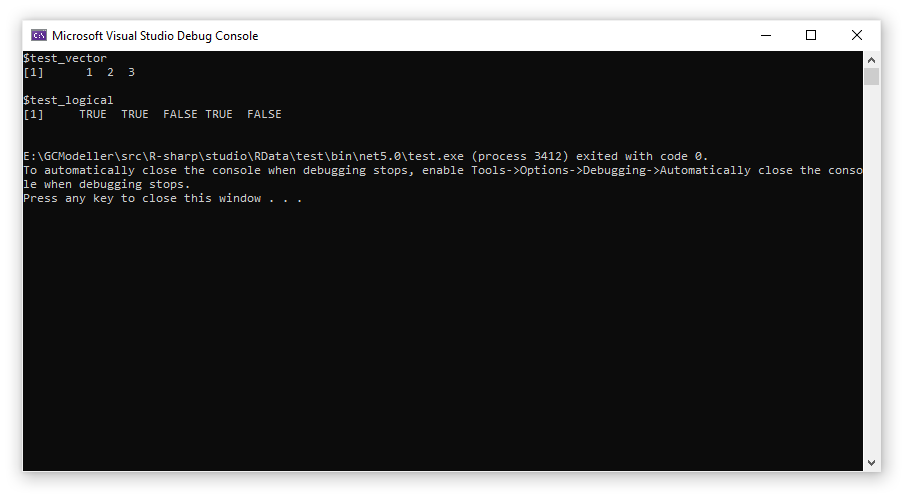### 反序列化list

Dim elements As RObject() = robj.value.data
Dim list As New list

For i As Integer = 0 To names.Length - 1
Next

Return list

If attrTags IsNot Nothing AndAlso attrTags.tag IsNot Nothing Then
Dim tag As RObject = attrTags.tag

If tag.characters = "names" Then
ElseIf tag.referenced_object IsNot Nothing AndAlso tag.referenced_object.characters = "names" Then
End If
End If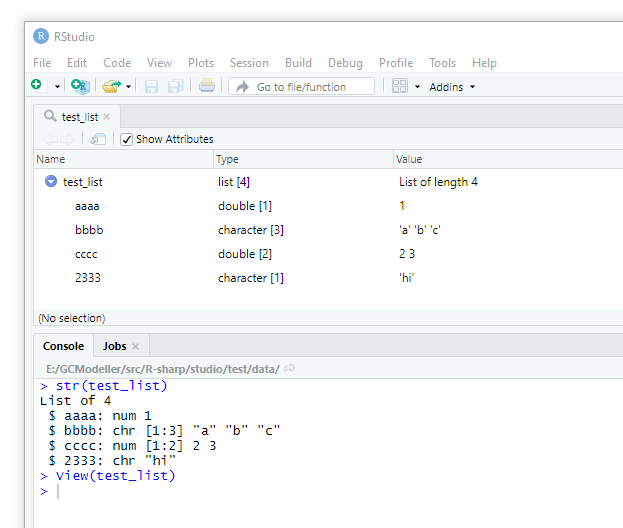### 反序列化dataframe

Public Shared Function IsPairList(robj As RObject) As Boolean
Dim attrs As RObject = robj.attributes

If attrs Is Nothing Then
If robj.info.type Like ConvertToR.elementVectorFlags Then
Return False
Else
Return True
End If
End If

Return False
End If

Return True
End Function

<Extension>
Private Function CreateRTable(robj As RObject) As dataframe
Dim columns As RObject() = robj.value.data
Dim colnames As String() = robj.readColumnNames
Dim vector As vector
Dim table As New dataframe With {
.columns = New Dictionary(Of String, Array),
}

For i As Integer = 0 To colnames.Length - 1
vector = columns(i).CreateRVector

If vector.factor Is Nothing Then
table.columns(colnames(i)) = vector.data
Else
table.columns(colnames(i)) = factor.asCharacter(vector)
End If
Next

Return table
End Function## 获取所有符号列表

Public Class RList

Public ReadOnly Property nodeType As ListNodeType
Get
If data Is Nothing AndAlso CAR Is Nothing AndAlso CDR Is Nothing Then
Return ListNodeType.NA
ElseIf Not data Is Nothing Then
Return ListNodeType.Vector
Else
End If
End Get
End Property

End Class
• 所有的属性值都是空值，则是空值NA状态
• 当data属性值不为空的时候，CAR和CDR两个属性节点一定为空值。这个时候的RObject是二叉树中的叶子节点，仅存储有向量数据
• 当data属性值为空的时候，CAR和CDR二者肯定有一个属性不为空值。这个时候RObject节点为一个链表中的链接节点

''' <summary>
''' Pull all R# object from the RData linked list
''' </summary>
''' <param name="rdata"></param>
''' <returns></returns>
'''
<Extension>
Private Function PullRObject(rdata As RObject, list As Dictionary(Of String, Object)) As Object
Dim value As RList = rdata.value
Dim car As RObject = value.CAR

If value.nodeType = ListNodeType.NA Then
Return Nothing
ElseIf value.nodeType = ListNodeType.Vector Then
' 已经没有数据了，结束递归
If RObjectSignature.IsPairList(rdata) Then
Return rdata.CreatePairList
ElseIf RObjectSignature.IsDataFrame(rdata) Then
Return rdata.CreateRTable
Else
Return rdata.CreateRVector
End If
Else
' CAR为当前节点的数据
' 获取节点数据，然后继续通过CDR进行链表的递归访问
Dim current As Object = PullRObject(car, list)
Dim currentName As String = rdata.tag.characters
Dim CDR As RObject = value.CDR

' pull an object

If CDR Is Nothing Then
Return current
Else
' 产生一个列表
Return PullRObject(CDR, list)
End If
End If
End Function

• NA状态的时候，已经到了叶节点处，并且叶节点中没有存储任何数据。则直接返回空值，退出整个递归
• 处于Vector状态的时候，也属于访问到了整个链表中的叶节点处。但是当前的RObject节点之中存储有数组或者向量数据，所以从这里开始我们可以进行上面所描述的向量类型，列表类型以及数据框类型的反序列化读取操作
• 最后，处于LinkedList链表状态的时候，说明在当前的RObject节点之后还存在有其他的符号对象值。我们在读取完当前节点的对象值之后，还需要访问CDR节点的值，进行整个二叉树链表的递归访问。

## R#脚本DEMO测试

<ExportAPI("readRData")>
Public Function parseRData(file As String) As Object
Using buffer As Stream = file.Open(FileMode.Open, doClear:=False, [readOnly]:=True)
Dim obj As Struct.RData = Reader.ParseData(buffer)
Dim symbols As list = ConvertToR.ToRObject(obj.object)

Return symbols
End Using
End Function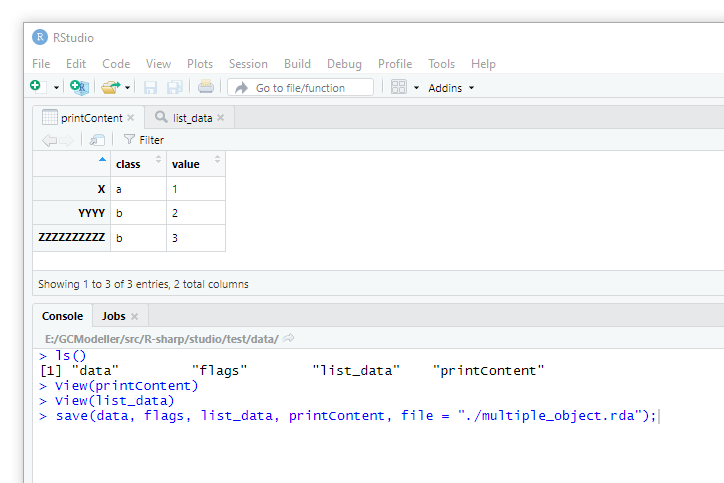list = base::readRData("multiple_object.rda");

print("get all symbols in target RData dataset:");
print(names(list));

print("===============================================");

for(name in names(list)) {
print("symbol name:");
print(name);

cat("\n");

if (typeof list[[name]] is "data.frame") {
print(list[[name]]);
} else {
str(list[[name]]);
}

cat("\n\n");
}Latest posts by 谢桂纲 (see all)

### Order by Date Name Attachments

#### 4 Responses

1.best service to buy instagram likes says:

This design is spectacular! You most certainly know how to keep a reader amused.
Between your wit and your videos, I was almost moved to start my own blog (well,
almost…HaHa!) Wonderful job. I really enjoyed what you had to say,
and more than that, how you presented it. Too cool!

来自瑞典
2.UK Teeth Whitening says:

Great content! Keep up the good work!

来自荷兰
•xie guigang says:

Hi, thanks

来自江苏
3. […] RData数据集反序列化至R#环境之中 […]

来自中国
1.2.3.查询字符串以["起始，以"]结尾： SELECT * FROM biodeepdb_full.metabolite WHERE INSTR(name, '["') = 1 AND INSTR(name, '"]') = LENGTH(name) - LENGTH('"]') + 1 ORDER…

4.删除mysql表中重复的行数据  delete t1 from metabolite t1 where t1.id in (select t2.id from (select id, name`, formula, hashcode2, row_number() over(partition…

5.